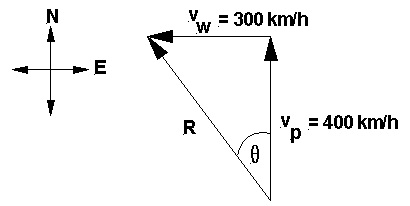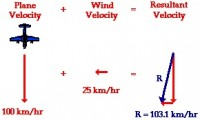# Vector Addition/Subtraction Calculator and Vector Component ResolverIn the realm of Physics and Math, one of the most useful fundamental concepts studied is how to work with vectors.  Vectors are measurements associated with direction and are used in the fields of construction, air traffic control, nautical navigation, and general travel/GPS calculations.

Whether you are adding, subtracting, or decomposing force vectors, velocity vectors, position vectors, rays and vectors defined by cartesian or polar coordinates, here are some great online based vector calculation tools that illustrate the skills taught in both physics and trigonometry/pre-calculus:

# Solving Force Vectors for Objects on a Ramp or Inclined Plane

• ## Inclined plane force calculator and problem solver

helps solve the problem, while showing all the steps in the various calculations and displaying a fully labeled free body diagram.